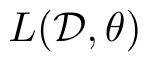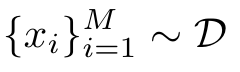# How to efficiently compute gradient for each training sample?

Hi folks,

There is a problem that has bothered me for quite a long time. Assume we are minimizing a loss functionparameterized by, on samplesusing SGD, where M is the mini-batch size. Since the PyTorch autograd can only be implicitly created for scalar outputs, I am wondering if there is any efficient way to compute the gradient for each sample, i.e.,, without setting the batch size equals to 1 and compute in a for loop (which is too slow)?

The shape of loss is (B, )，which is the batch size

1 Like

Hi Zhaomang,

Thanks so much for your reply! If the loss is set to be a non-scaler, i.e., the sample loss:

``````loss = F.cross_entropy(predictions, results, reduction='none')
``````

I’m still confused about how to get the gradient on each sample, if I run the following code:

``````for params in model.parameters():
``````

I expect to get `B` times the number of tensors returned in the mini-batch case, since there should be an individual result for each sample.

In addition, it would be very helpful if you could point me to the document of Tensor.backward() function (if any), so that I can learn more on this. Thanks again!

If you just have conv/linear layers, you could use this – https://github.com/cybertronai/autograd-hacks#per-example-gradients

1 Like

But if you need to obtain the gradient on each sample, you can set

Are you going to extend this to other popular layer types, e.g. ReLu and batchnorm? This would make it useful for most widely used architectures such as Resnets

It should work for those architectures as well. What’s missing is support for other layers with trainable parameters, (ie the multiheadattention layer)

Not with batch norm. If I understood your implementation correctly, layers with batch normalization are just skipped:

``````_supported_layers = ['Linear', 'Conv2d']

if layer_type not in _supported_layers:
continue
``````

Which means that for batch normalization layer (which is trainable), grad1 will not be created.

Ah right, the gamma, beta parameters. It seems feasible to extend computation explained here to per-example computation https://kratzert.github.io/2016/02/12/understanding-the-gradient-flow-through-the-batch-normalization-layer.html

Can the current version of autograd-hacks still be used if I apply other activation functions such as Sigmoid / tanh / ReLU? Thanks.

Yes, any activation function that works with PyTorch autograd should also work here